# Third Chapter Lesson-12: Universal Gates(NAND,NOR) & Exclusive Gates(XOR,XNOR)

## At the end of this lesson-

• You will be able to explain compound gate.
• You will be able to describe the Universal gates.
• You will be able to describe NAND & NOR gates in details.
• You will be able to describe the Exclusive gates in details.
• You will be able to describe X-OR & X-NOR gates in details.

## Compound/Composite Gates:

A gate that is created using two or more basic gates is called a composite or compound gate. For example-

AND Gate +NOT Gate = NAND Gate,  OR Gate + NOT Gate = NOR Gate. Composite gates are two types. They are-

• Universal Gate (NOR & NAND)
• Exclusive Gate (X-OR & X-NOR)

## The “Universal” Gates:

The gate which can implement all the basic gates (AND, OR, NOT) as well as any gate and any circuit is called a universal gate. NAND and NOR gates are called universal gates. This is because only NAND gate or only NOR gate can implement all the basic gates (AND, OR, NOT) as well as any gate and any circuit.These gates are widely used in digital circuits as these cost less to make.Fig: Universal Gates

## Logic NOR Gate:

The Logic NOR Gate is a compound gate which is combination of the logic OR gate and an NOT gate connected together in series. If the output of the OR gate is propagated to the NOT gate, then the NOR gate is available. That is, by reversing the output of the OR gate, the output of the NOR gate is obtained.

The inclusive NOR  gate has an output that is normally at logic level “1” and only goes “LOW” to logic level “0” when ANY of its inputs are at logic level “1”. The Logic NOR Gate is the reverse or “Complementary” form of the inclusive OR gate we have seen previously.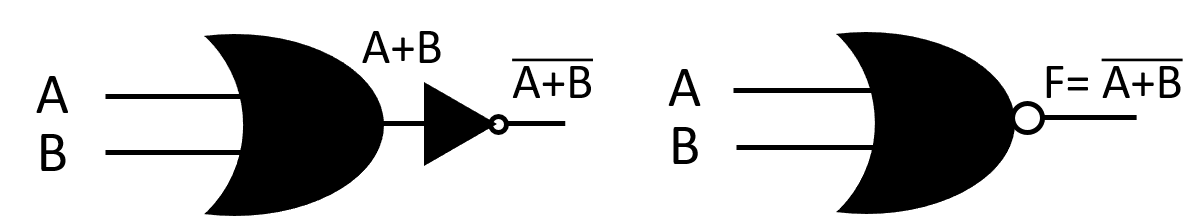Fig: OR Gate + NOT Gate = NOR Gate

The NOR gate has two or more input lines and only one output line. NOR gate is also known as compound gate and universal gate.

### 3-input NOR Gate: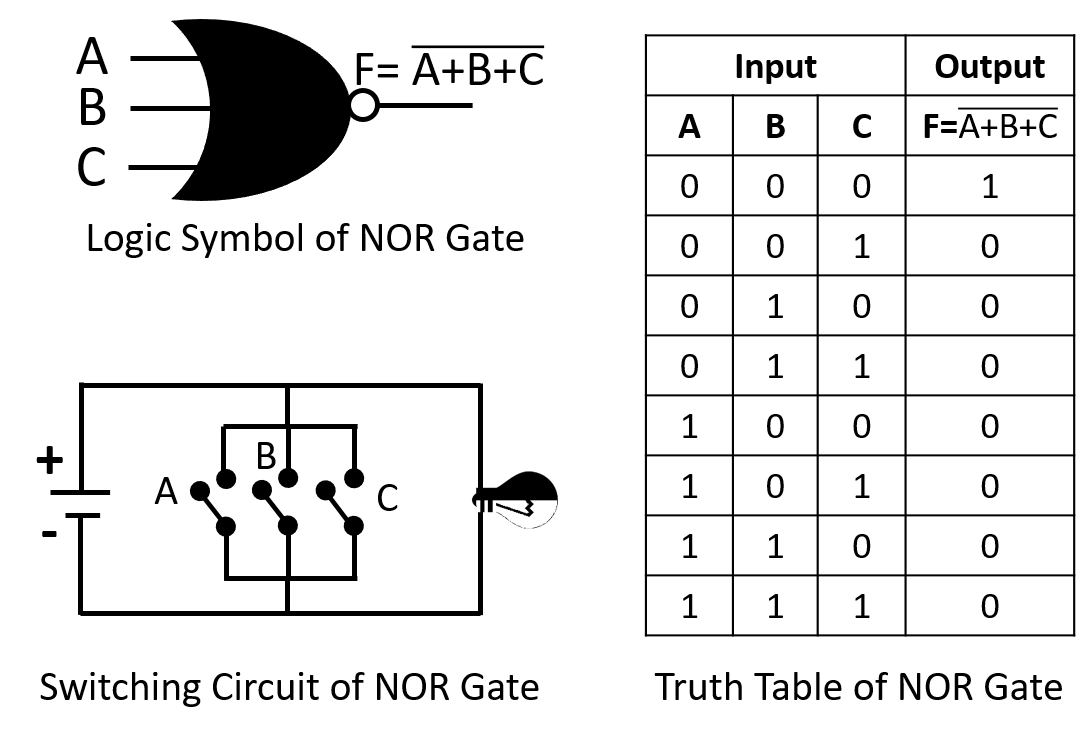### Logic NAND Gate:

The Logic NAND Gate is a composite gate which is combination of a logic AND gate and a NOT gate connected together in series. If the output of the AND gate is propagated to the NOT gate, then the NAND gate is available. That is, by reversing the output of the AND gate, the output of the NAND gate is obtained.

The NAND gate has an output that is normally at logic level “1” and only goes “LOW” to logic level “0” when ALL of its inputs are at logic level “1”. The Logic NAND Gate is the reverse or “Complementary” form of the AND gate we have seen previously.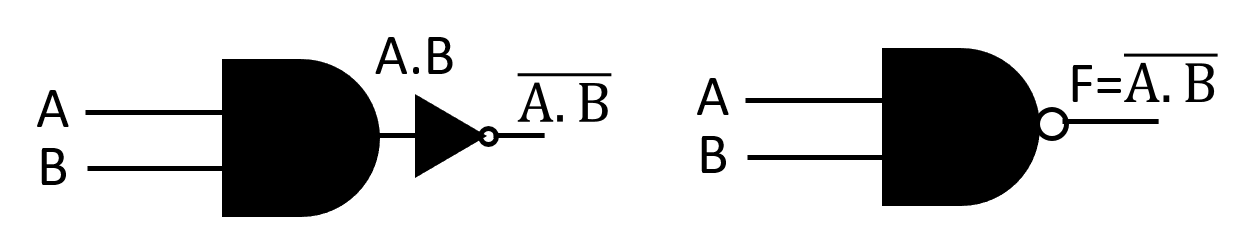Fig: AND Gate + NOT Gate = NAND Gate

The NAND gate has two or more input lines and only one output line. NAND gate is also known as compound gate and universal gate.

### 2-input Logic NAND Gate: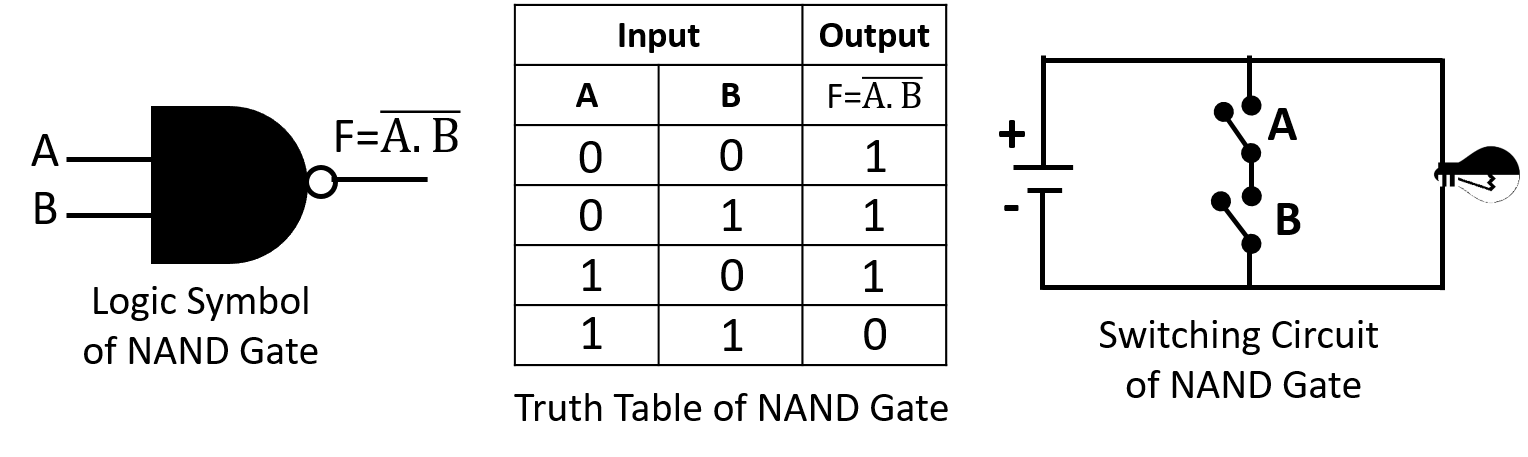## Exclusive Gates:

These two “hybrid” logic gates are called the Exclusive-OR (Ex-OR) Gate and its complement the Exclusive-NOR (Ex-NOR) Gate.

### Exclusive-OR Gate:

The Exclusive OR Gate is abbreviated as the X-OR Gate. This is a compound gate made up of AND, OR and NOT gates. The output signal is obtained by comparing different input bits through this gate. The output is “1” when there are an odd number of 1’s in the inputs. otherwise it is 0. The X-OR operation is represented by ⊕ simbol.

The X-OR gate has two or more input lines and only one output line. The X-OR gate is also known as the compound gate and the special gate. The Exclusive-OR logic gate is very useful circuit that can be used in many different types of computational circuits. It is used extensively in building arithmetic logic circuits, computational logic comparators and error detection circuits.

### 2-input Ex-OR Gate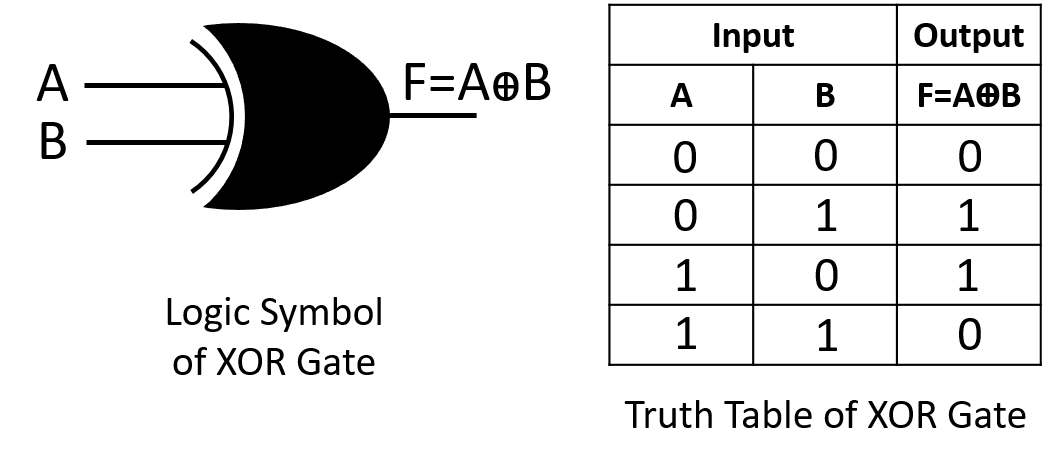The following Boolean Expression can be written from the above truth table of XOR gate using SOP method-

F = A´ B +

Implementation of the above Boolean Expression-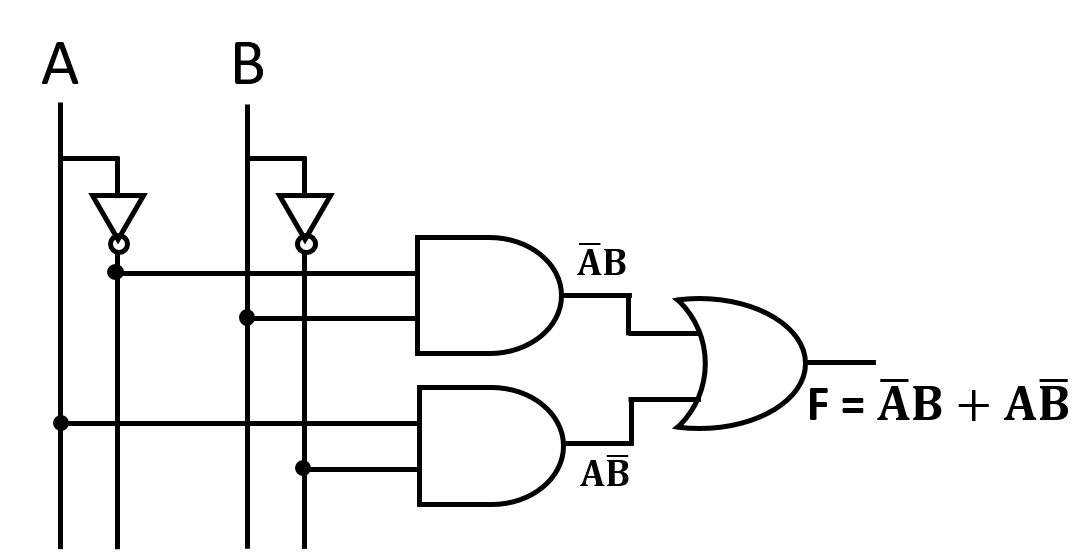Fig: Implementation of XOR gate using Basic gates

### 3-input Ex-OR Gate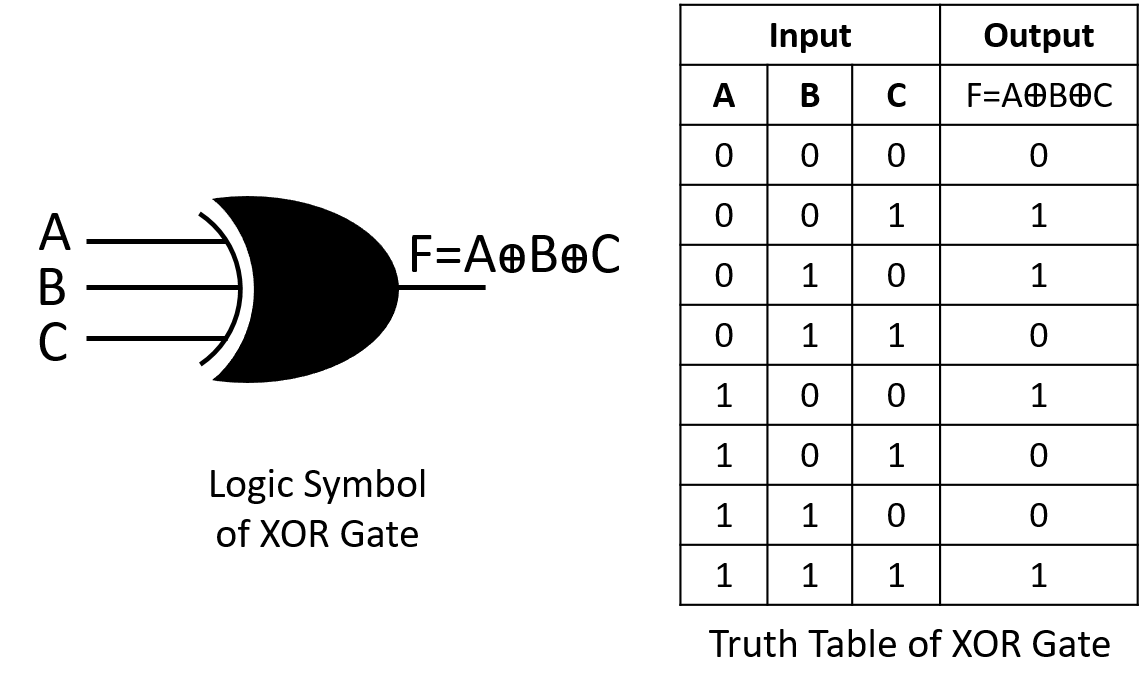The following Boolean Expression can be written from the above truth table of XOR gate using SOP method-

F = A´B´C + B + AB´C´ + ABC

Implement the above Boolean Expression.

### Exclusive-NOR Gate:

The Exclusive NOR Gate is abbreviated as the X-NOR Gate. It is combination of the Exclusive-OR gate and the NOT gate connected together in series that is also the reverse or complementary of the Exclusive-OR gate. This is a compound gate made up of AND, OR and NOT gates. If the output of the X-OR gate is propagated to the NOT gate, then the X-NOR gate is available. That is, by reversing the output of the X-OR gate, the output of the X-NOR gate is obtained. An even number of logic “1’s” on its inputs gives a logic “1” at the output, otherwise is at logic level “0”. The logic symbol for an Exclusive-NOR gate is simply an Exclusive-OR gate with a circle or “inversion bubble”, ( ο ) at its output to represent the NOT function.Fig: XOR Gate + NOT Gate = XNOR Gate

The X-NOR gate has two or more input lines and only one output line. The X-NOR gate is also called the composite gate and the special gate.

### 2-input Ex-NOR Gate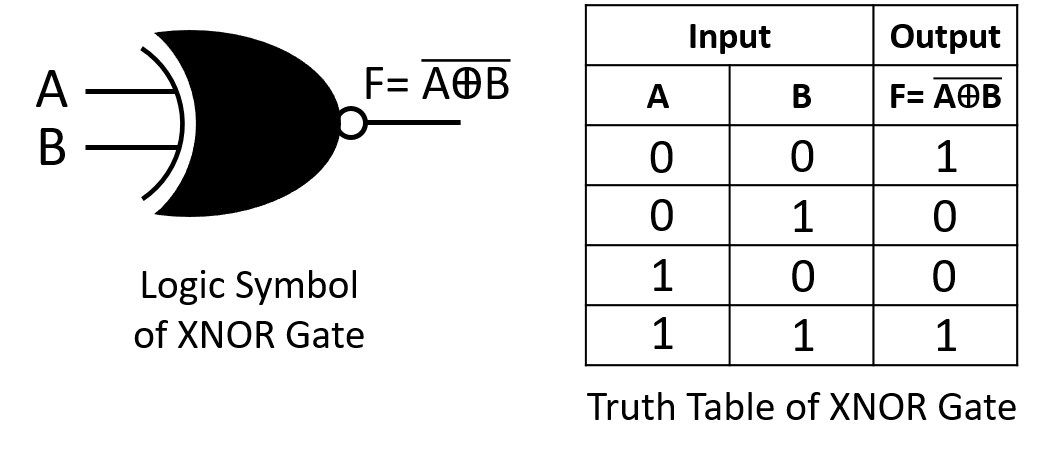The following Boolean Expression can be written from the above truth table of XNOR gate using SOP method-

F = A´ + AB

Implementation of the above Boolean Expression-Fig: Implementation of XNOR gate using Basic gates

### 3-input Ex-NOR Gate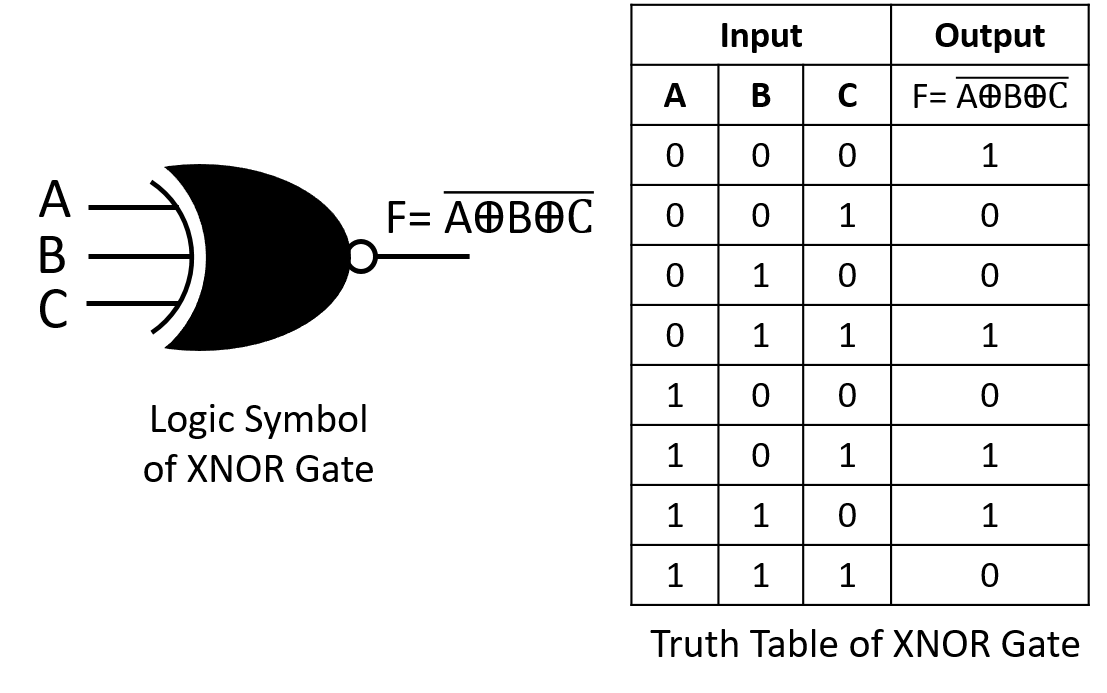The following Boolean Expression can be written from the above truth table of XNOR gate using SOP method-

F = A´B´C´ + A´BC + AC + ABC´

Implement the above Boolean Expression.

## Lesson Evaluation-

Knowledge Based Questions:

• a. What is compound gate?
• a. What is universal gate?

Comprehension Based Questions:

• b. Which gates are called universal gate?
• b. “NOR gate is called universal gate”-Explain.
• b. “NAND gate is called universal gate”-Explain.
• b. “X-OR gate is a combinational logic gate of all basic gates”-Explain.
• b. Which basic gates are used in implementing X-OR gate? Explain.
• b. “X-NOR gate is a combinational logic gate of all basic gates”-Explain.
• b. Which basic gates are used in implementing X-NOR gate? Explain.
• b. What will be the output of XOR gate if an input is 1 and another is A?
• b. Explain the advantages of XOR over OR gate.
• b. Bulb is not lightened if two switches are ON- Explain in case of logic gate.

Creative Questions:

According to the stem answer the following questions: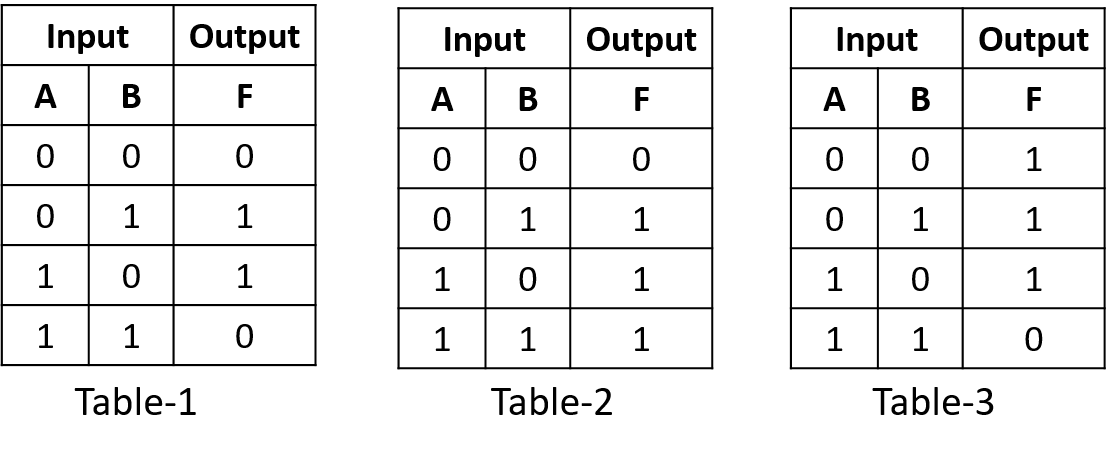c) Prove that the truth table-3 represents the NAND gate.

d) Draw the logic circuit of table-1 in case of three inputs.

According to the stem answer the following questions:c) Fig-1 and Fig-2 are which type of gate? Explain

d) Which gate is created when the output of Fig-3 flows in the NOT gate? Argue for the answer.

According to the stem answer the following questions: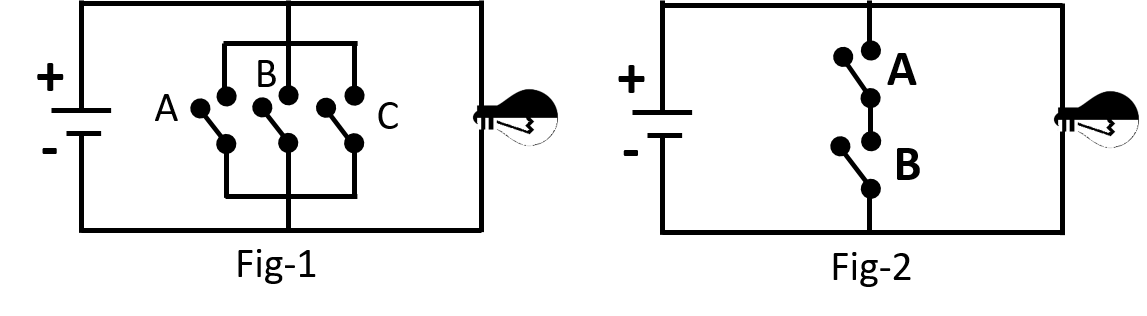c) When the two switches in Fig-2 are connected in parallel with each other it turns into basic gate- explain.

d) The bulb is not lighten when any one switch of Fig-1 switched ON- Analyze.

Multiple Choice Questions:

1. How many compound gates can be created using basic gates?

a) 2   b) 4     c) 6    d) 8

2. Which one is compound gate?

a) OR      b) AND       c) NAND       d) NOT

3. Which gate makes XNOR gate connecting with XOR?

a) OR      b) AND       c) NAND       d) NOT

4. Output of NAND gate will be ‘1’ if —

i. All inputs are 1      ii. Any one input is 1      iii. All inputs are 0

Which one is correct?

a) & ও ii   b) i & iii   c) ii & iii   d) i, ii & iii

5. Output of NOR gate will be ‘0’ if —

i. All inputs are 0      ii. Any one input is 1      iii. All inputs are 1

Which one is correct?

a) & ও ii   b) i & iii   c) ii & iii   d) i, ii & iii

6. Output becomes 1 even though both inputs are 0 in—- gate.

i. XOR    ii. NOR     iii. XNOR

Which one is correct?

a) & ও ii   b) i & iii   c) ii & iii   d) i, ii & iii

Written by,